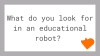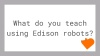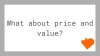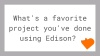GLOBAL SITE AUSTRALIAN SITE
Select Page

# Lesson 9 Worksheet 9.4 – Light following

Write the following program to have the Edison robot follow light from a torch/flashlight.Shine a torch/flashlight at Edison and the robot will drive towards the light.

This program performs a calculation between two variables. In the calculate word icon the variable Right_Light is subtracted from the variable Left_Light. The result (answer) is placed back into the variable Right_Light. Let’s see what the results would look like:

 Right_Light Left_Light Calculation Result Torch in right 200 100 200 – 100 = -100 Torch on left 100 200 100 – 200 = +100

From the above you can see that when the torch is to the right the result is below zero (negative number). When the torch is to the left the result is above zero (positive number).

The If icon asks: Is the result less than zero? If true the robot drives left (towards the light), if false the robot drives right (towards the light).

What wold happen if you changed the ‘less than’ symbol (<) to a greater than symbol (>)?

______________________________________________________________________________________________________________________________________________

______________________________________________________________________________________________________________________________________________

• ### Keep up with Edison

• This field is for validation purposes and should be left unchanged.